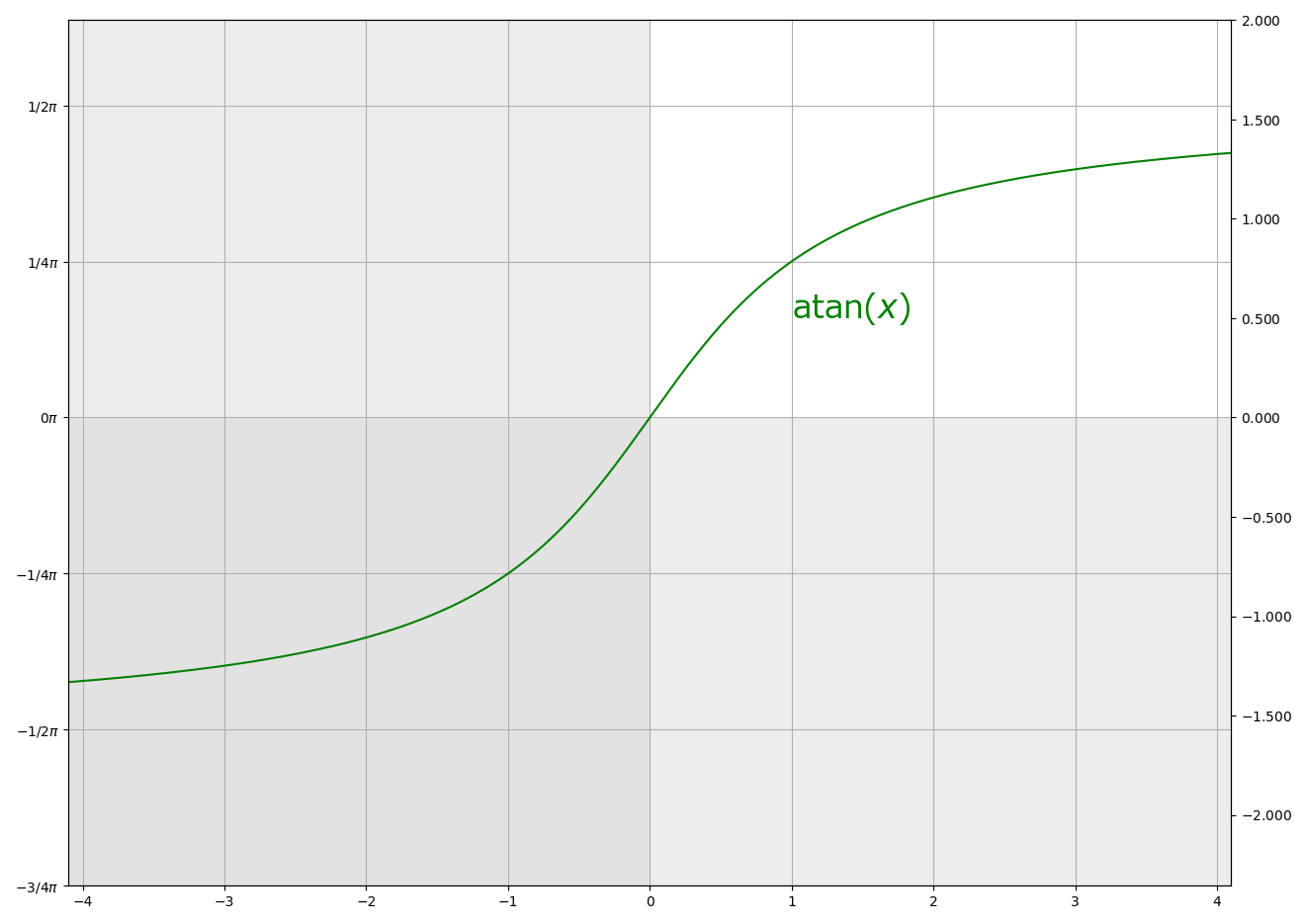# (ffmpeg-utils)3. Expression Evaluation¶

doc

Expression Evaluation

(ffmpeg-utils)3. Expression Evaluation (2)

Official documentation says:

When evaluating an arithmetic expression, FFmpeg uses an internal formula evaluator, implemented through the libavutil/eval.h interface.

An expression may contain unary, binary operators, constants, and functions.

Two expressions expr1 and expr2 can be combined to form another expression "expr1;expr2". expr1 and expr2 are evaluated in turn, and the new expression evaluates to the value of expr2.

The following binary operators are available: +, -, *, /, ^.

The following unary operators are available: +, -.

## Constants¶

• PI

area of the unit disc, approximately 3.14

• E

$$\exp(1)$$ (Euler’s number), approximately 2.718

• PHI

golden ratio $$(1 + \sqrt{5}) / 2$$, approximately 1.618

## Functions¶

### Logical functions¶

Official documentation says:

if(x, y)

Evaluate x, and if the result is non-zero return the result of the evaluation of y, return 0 otherwise.

if(x, y, z)

Evaluate x, and if the result is non-zero return the evaluation result of y, otherwise the evaluation result of z.

ifnot(x, y)

Evaluate x, and if the result is zero return the result of the evaluation of y, return 0 otherwise.

ifnot(x, y, z)

Evaluate x, and if the result is zero return the evaluation result of y, otherwise the evaluation result of z.

not(expr)

Return 1.0 if expr is zero, 0.0 otherwise.

.

between(x, min, max)

Return 1 if x is greater than or equal to min and lesser than or equal to max, 0 otherwise.

eq(x, y)

Return 1 if x and y are equivalent, 0 otherwise.

gt(x, y)

Return 1 if x is greater than y, 0 otherwise.

gte(x, y)

Return 1 if x is greater than or equal to y, 0 otherwise.

lt(x, y)

Return 1 if x is lesser than y, 0 otherwise.

lte(x, y)

Return 1 if x is lesser than or equal to y, 0 otherwise.

.

isinf(x)

Return 1.0 if x is +/-INFINITY, 0.0 otherwise.

isnan(x)

Return 1.0 if x is NAN, 0.0 otherwise.

Ffmpeg does not claim “confusing logical values and numbers is catastrophically dangerous” as in some modern programming languages. So, you can mix the results of logical values into the arithmetic operations as they are:

[me@host: ~]$ffplay video.mkv -af "volume='lte(t, 2) * 0.1 + gt(t, 2)':eval=frame"  ### bitand(x, y), bitor(x, y)¶ Official documentation says: bitand(x, y), bitor(x, y) Compute bitwise and/or operation on x and y. The results of the evaluation of x and y are converted to integers before executing the bitwise operation. Note that both the conversion to integer and the conversion back to floating point can lose precision. Beware of unexpected results for large numbers (usually $$2^{53}$$ and larger). [me@host: ~]$ # The following two will have the same result:
[me@host: ~]$ffplay video.mkv -vf "lutyuv=y='bitand(val, 0xF0)'" [me@host: ~]$ ffplay video.mkv -vf "lutyuv=y='val - mod(val, 0x10)'"


### Trigonometric functions¶

Official documentation says:

cos(x)

Compute cosine of x.

sin(x)

Compute sine of x.

tan(x)

Compute tangent of x.[me@host: ~]$ffplay -f lavfi "aevalsrc='0.5 * sin(440 * 2 * PI * t)'" [me@host: ~]$ ffplay -f lavfi "aevalsrc='0.5 * cos(20 * t) * sin(440 * 2 * PI * t)'"


### Inverse trigonometric functions¶

Official documentation says:

acos(x)

Compute arccosine of x.

asin(x)

Compute arcsine of x.

atan(x)

Compute arctangent of x.

atan2(x, y)

Compute principal value of the arc tangent of y/x.### sgn(x)¶

Official documentation says:

sgn(x)

Compute sign of x.



### ceil(expr), floor(expr), trunc(expr), round(expr)¶

Official documentation says:

ceil(expr)

Round the value of expression expr upwards to the nearest integer. For example, ceil(1.5) is 2.0.

floor(expr)

Round the value of expression expr downwards to the nearest integer. For example, floor(-1.5) is -2.0.

trunc(expr)

Round the value of expression expr towards zero to the nearest integer. For example, trunc(-1.5) is -1.0.

round(expr)

Round the value of expression expr to the nearest integer. For example, round(1.5) is 2.0.Note

Note that the behavior of the “round” function may differ from that of your favorite programming language (ex. “round” of Python).



### random(x)¶

Official documentation says:

random(x)

Return a pseudo random value between 0.0 and 1.0. x is the index of the internal variable which will be used to save the seed/state.